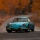Home
IT Knowledge
Inspiration
Languages
EN

# Java - replace first 2 characters in string

0 points
Created by:Vadim-Kotiv
294

In this article, we would like to show you how to replace the first 2 characters in string in Java.

Quick solution:

``````String text = "ABCD";
String replacement = "xy";
String result = replacement + text.substring(2);

System.out.println(result);  // xyCD``````

or:

``````String text = "ABCD";
String replacement = "xy";
String result = replacement.concat(text.substring(2));

System.out.println(result);  // xyCD``````

or:

``````String text = "ABCD";
String replacement = "xy";
String result = text.replaceAll("^.{2}", replacement);

System.out.println(result);  // xyCD``````

## 1. Practical example using String `substring()`

### 1.1 With `+` operator

In this example, we will take the last `2` characters from the original string and add them to the end of the replacement.

``````public class Example {

public static void main(String[] args) {
String text = "ABCD";
String replacement = "xy";
String result = replacement + text.substring(2);

System.out.println(result);  // xyCD
}
}``````

### 1.2 With `concat()` method

This approach is equivalent to the above one. Instead of `+` operator we concatenate the strings using `concat()` method.

``````public class Example {

public static void main(String[] args) {
String text = "ABCD";
String replacement = "xyz";
String result = replacement.concat(text.substring(3));

System.out.println(result);  // xyCD
}
}``````

## 2. Practical example using String `replaceAll()` with regex pattern

In this example, we use string `replaceAll()` with `"^.{2}"` regex to replace the first 2 characters in the `text` string.

Regex explanation:

• `^` - matches the beginning of the string,
• `.` - matches any character except linebreaks,
• `{2}` - matches the specified quantity of the previous token (in our case the `.`).
``````public class Example {

public static void main(String[] args) {
String text = "ABCD";
String replacement = "xy";
String result = text.replaceAll("^.{2}", replacement);

System.out.println(result);  // xyCD
}
}``````

Note:

Regular expressions are slower than `substring()` method.